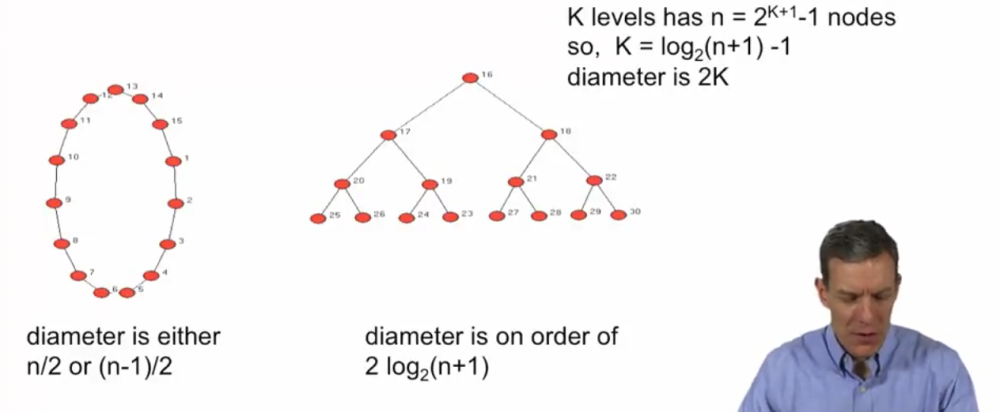# Diameter, Average Path Length¶

• How close are nodes:

• How long does it take to reach average node?

• How fast will information spread?

# Diameter¶

• Diameter - largest geodesic (largest shortest path)

• if unconnected, of largest component...
• Average path length

• less prone to outliers

One thing about diameter is it can be prone to outliers. It could be that happen to be one pair of nodes which are very far from each other, but a lot of the other ones are relatively well connected to each other. So average path length is going to be a different measure than the diameter inside of the maximum geodesic. It's goting to be the average geodesic in network.In the tree network, there are 3 levels: 3 differnet links(16-17, 17-20, 20-25)

The tree network diameter is 6, node 25 to node 30

# Small average path length and diameter¶

• Milgram(1967) letter experiments

• median 5 for the 25% that made it
• Co-Authorship studies

• Grossman(1999) Math mean 7.6, max 27
• Newman(2001) Physics mean 5.9, max 20
• Goyal el al(2004) Economics mean 9.5, max 29
• WWW

• Adamic, Pitkow(1999) - mean 3.1 (85.4% possible of 50M pages)

• Backstrom et al(2012) - mean 4.74 (721 million users)

# Neighborhood and Degree¶

• Neighborhood: $N_i(g)$ = {j | ij in g}, usual convention ii not in g

• Degree: $d_i$ = # $N_i(g)$

• The degree of node i is just simply counting up how many neighbors a given node i has in the network g.

# Erdos-Renyi (1959, 1960) Random Graphs¶

• each link is formed independently with some probability p

• Serves as a benchmark "G(n, p)", graph on n nodes with a probability p

• It's a very important benchmark in understanding things, because if we see some network out there in the real world , if we know what the properties of a random network of nodes with some probability p was, then we can compare the real world network that we see to this benchmark network and say does it look like something systematic is going on? Does this network look systematically different than if nature had just picked links at random? Or does it look like something is systematically different?

# Sequences of Networks¶

• Links are dense enough so that network is connected almost surely:

• $d(n) \ge (1+\epsilon)log(n)$ some $\epsilon \ge 0$
• $\frac{d(n)}{n} \rightarrow 0$: network is not too complete

# Theorem on Network Structure¶

If $d(n) \ge (1+\epsilon)log(n)$ some $\epsilon \ge 0$ and $\frac{d(n)}{n} \rightarrow 0$:

then for large n, average path length and diameter of this G(n, p) graph are approximately proportional to $\frac{log(n)}{log(d)}$

$$\frac{AvgDist(n)}{log(n) / log(d(n))} \rightarrow ^p 1$$

(Proven for increasingly general models: Erdos-Renyi 59 - Moon and Moser 1966, Bollobas 1981; Chung and Lu 01; Jackson 08;...)